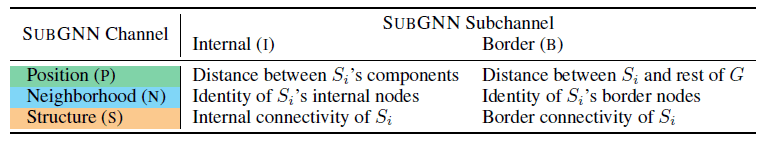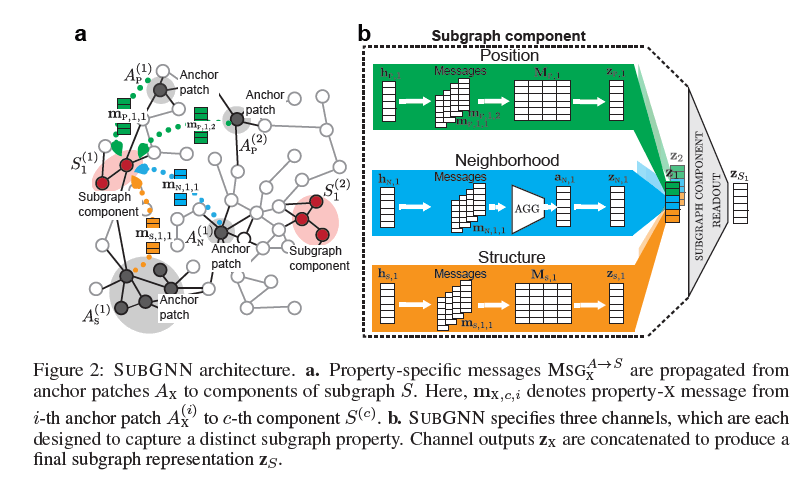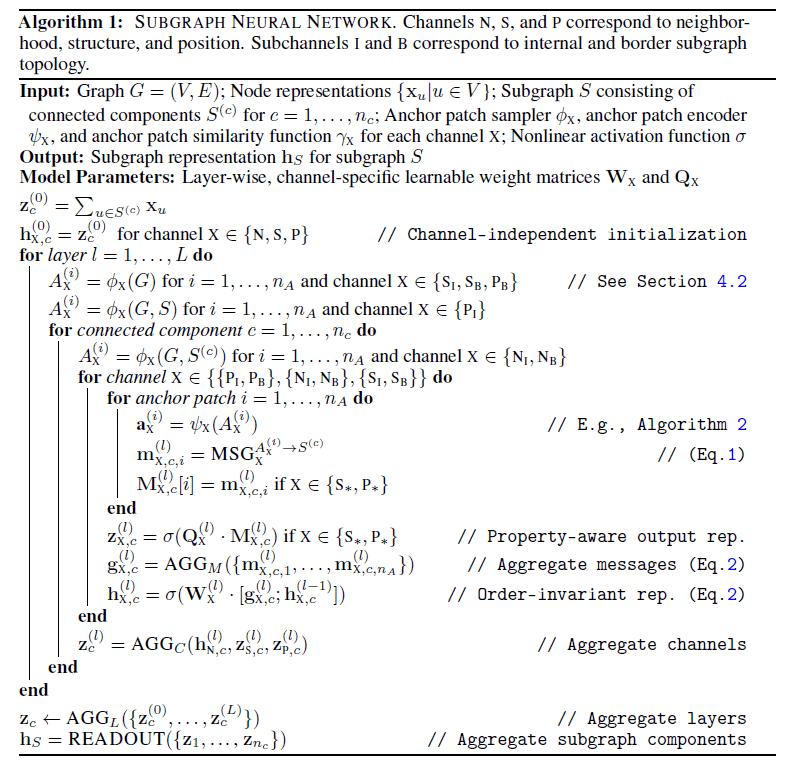[TOC]

子图表示学习

摘要

Sub2Vec[PAKDD’18]

• 标签：随机游走
• 摘要：Sub2Vec通过随机游走来捕获子图结构和领域两个角度的特征，在结构角度将随机游走的边权重定义为顶点度数与子图大小的比值，来避免子图规模带来的影响。Sub2Vec还借鉴了Paragraph2Vec的思想，在学习子图特征表示时保留相似（有着类似的node co-occurrence）子图间的距离
• 有无开源代码：https://github.com/bijayaVT/sub2vec

Sub-graph Contrast for Scalable Self-Supervised Graph Representation Learning[ICDM’20]

• 标签：PPR
• 链接：https://arxiv.org/abs/2009.10273v3
• 摘要：SUBG-CON将一个中心顶点邻域中PPR值topk的顶点作为子图，通过一个GNN将子图转换成特征表示。有了中心顶点和子图各自的特征表示后，进一步使用了对比学习：子图的表示作为中心顶点表示的正样本，将子图进行扰乱后作为负样本。因为正负样本实际都来自相同的子图，论文没有使用交叉熵损失函数，而是边缘损失。
• 有无开源代码：https://github.com/yzjiao/Subg-Con

笔记

SGRL[WWW投稿]

SGRL中通过对子图内顶点的特征表示做平均来得到子图的特征表示：

SubGNN[NIPS’20]• 位置

子图内顶点间的距离以及子图与图上其余顶点间的距离。

• 邻域

不同于传统意义上单个顶点的邻域，一个子图的邻域有内有外，内邻域也就是子图本身，而外邻域是子图内所有顶点的$k$阶邻居组成的顶点集合。子图内每个连通分量都会有对应的外邻域。

• 结构

子图的内结构为内部顶点的连通性，而外结构为将内部顶点与外邻域相连的边的集合。1. 在网络的每一层，对于每个通道$X\in\{N,S,P\}$，$N,S,P$分别指代邻域，结构和位置。从图$G$中随机采样若干个子图$\mathcal{A}_X=\{A_X^{(1)},\dots, A_X^{(n_A)}\}$

2. 对于子图$S$中的每个连通分量$S^{(c)}$，与采样的子图集$\mathcal{A}_X$中的每个子图$A_X$进行信息传递，其中$\gamma_X$为相似度函数，$a_X$为$A_X$的特征表示：

3. 将传递的信息转化为当前通道下当前连通分量的特征表示：

4. 第三步中得到的特征表示次序无关，适合网络中层与层之间的传播，但对于位置与结构这两个通道来说，这种做法会限制特征表示的学习能力。所以，这两个通道的特征表示学习采用另一种方式：

1. 对于每个连通分量$S^{(c)}$，将第$l$层学习到的三个通道的特征表示进行聚合，得到单个连通分量在这一层的特征表示，再将所有层的结果进行聚合，得到该连通分量通过网络学习到的特征表示。最后将子图内所有连通分量的特征表示进行聚合，就得到了子图的特征表示。• 位置

没看懂文中的表述，准备结合代码理解

• 邻域

内采样器从连通分量$S^{(c)}$中采样顶点，边界采样器从$S^{(c)}$中顶点的$k$阶邻居中采样顶点。

• 结构

内顶点和边界顶点使用相同的采样器，从图$G$中使用三角随机游走的形式采样连通分量。

• 位置

内和边界一样处理，以两个子图之间的最短距离来衡量相似度，最短距离越短则相似度越高。

• 邻域

与位置通道类似，不过对于内邻域$\gamma_{N_I}=1$而边界邻域$\gamma_{N_B}\le k$。

• 结构

结构通道的相似度是通过DTW，一个衡量两个时间序列之间相似度的指标。在论文中使用的序列是将子图中顶点的度按照大小进行排序形成的序列；

AQCL

AQCL在顶点之间的对比学习的基础上加入了顶点和簇的对比学习：

1. 顶点间对比：给点一个顶点$z$以及它对应的数据增强的正样本$z^+$和负采样得到的负样本$z^-$，则$z$的特征表示应该靠近$z^+$并且远离$z^-$。
2. 顶点与簇间对比：给定兴趣簇$Q$, $z$也应该靠近其中的正簇同时远离负簇。我的理解是，这里的兴趣类似于隐语义模型中的隐因子（latent factor），将用户的点击数据投影到特征空间时能够挖掘出其中隐藏的规律，例如用户A更喜欢点击与运动、数码相关的物品等，虽然实际中兴趣可能没有任何实质意义。

AQCL解决的是CTR预估中的冷启动问题，这也是它加入顶点与簇间对比的动机。在这个问题中，用户的点击行为符合长尾分布，如果以一个用户点击过的所构成的序列长度来衡量他的活跃程度，只有极少数用户会点击很多物品，绝大多数用户的点击序列都很短。传统的顶点间对比学习无法捕获顶点邻域中的信息。一个直观的想法是，对于这些非活跃用户，可以让他们在特征空间中利用活跃用户的信息，来弥补交互数据的不足。